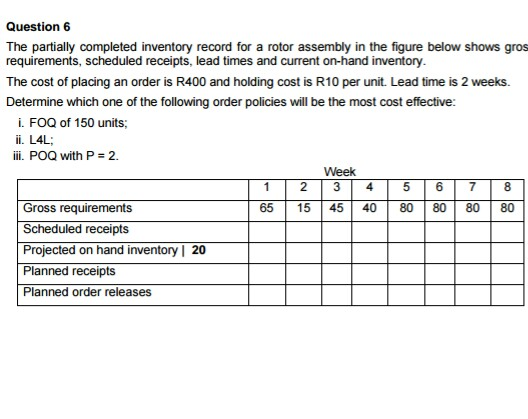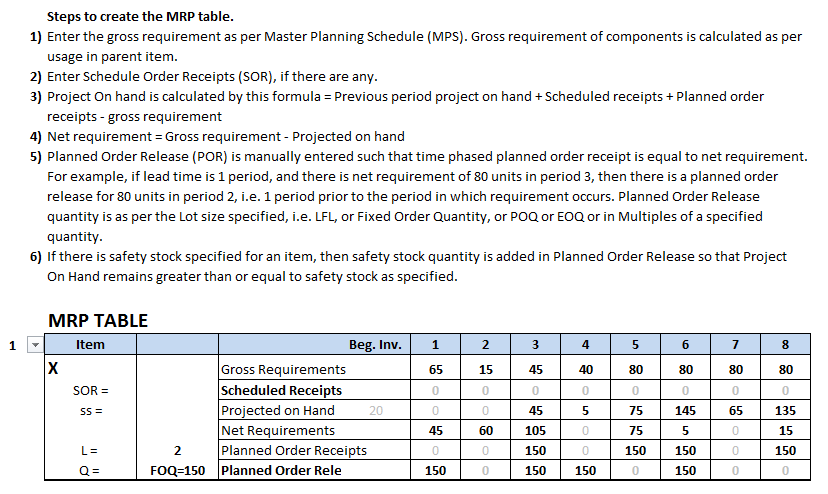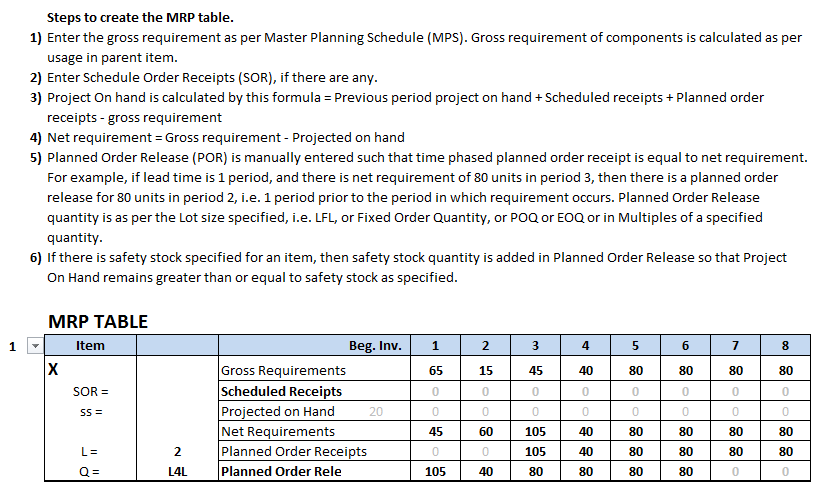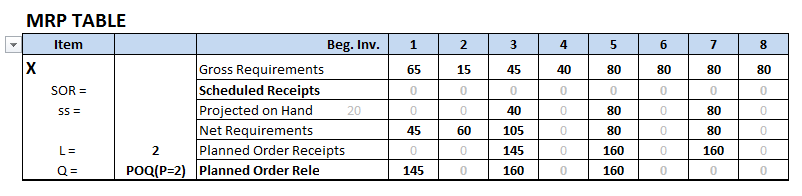# Question & Answer: The partially completed inventory record for a rotor assembly in the figure below…..The partially completed inventory record for a rotor assembly in the figure below shows gros requirements, scheduled receipts, lead times and current on-hand inventory. The cost of placing an order is R400 and holding cost is R10 per unit. Lead time is 2 weeks. Determine which one of the following order policies will be the most cost effective: i. FOQ of 150 units: ii. L4L: iii. Pod with P = 2.

i) FOQ of 150 units

Don't use plagiarized sources. Get Your Custom Essay on
Question & Answer: The partially completed inventory record for a rotor assembly in the figure below…..
GET AN ESSAY WRITTEN FOR YOU FROM AS LOW AS \$13/PAGETotal cost = Holding cost + Ordering cost = (45+5+75+145+65+135)*10 + 4*400 = 6300

ii) L4L Ordering policyTotal cost = Holding cost + Ordering cost = 0 + 6*400 = 2400

iii) POQ with P = 2 weeksTotal cost = Holding cost + Ordering cost = (40+80+80)*10 + 3*400 = 3200

We see that the total cost is lowest in case of L4L . Therefore L4L policy is the most cost effective.